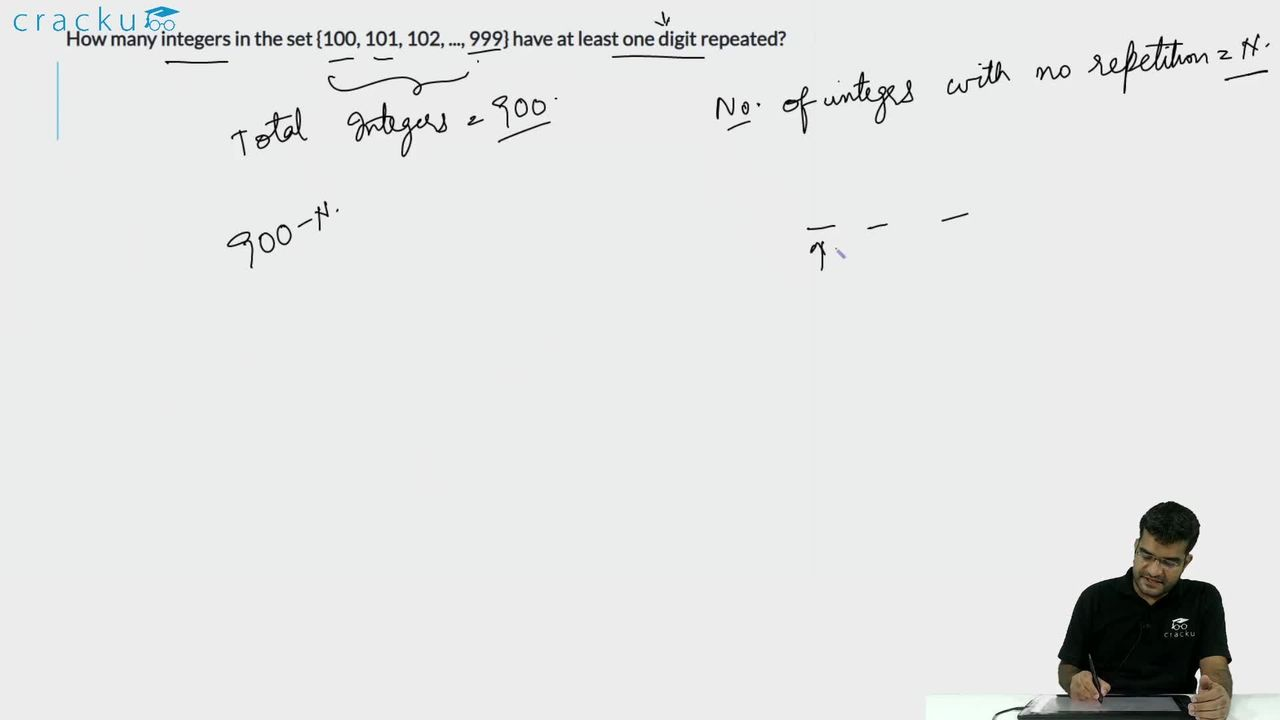Question 60

# How many integers in the set {100, 101, 102, ..., 999} have at least one digit repeated?

Solution

Total number of numbers from 100 to 999 = 900

The number of three digits numbers with unique digits:

_ _ _

The hundredth's place can be filled in 9 ways ( Number 0 cannot be selected)

Ten's place can be filled in 9 ways

One's place can be filled in 8 ways

Total number of numbers = 9*9*8 = 648

Number of integers in the set {100, 101, 102, ..., 999} have at least one digit repeated = 900 - 648 = 252

### View Video Solution